## An Update to the HRC-I MCP QE Model

### Abstract

We present an updated model (N0008) for the HRC-I MCP quantum efficiency. This model, released in the December 2010 CALDB, is based on cross-calibration with the HRC-S and ACIS and includes the effects of updated response models for those instruments. The calibration sources used are HZ 43 (soft band), PKS 2155-304 (medium band) and G21.5-0.9 (hard band).

### 1. Introduction

The normalization of the in-flight HRC-I microchannel plate (MCP) quantum efficiency (QE) model is determined by cross-calibration with the HRC-S/LETG and ACIS using common calibration sources. In the past two years, the HRMA effective area model, ACIS contamination model, and HRC-S QE model have been updated, prompting us to update the HRC-I QE model. This page documents the creation of this newest QE model, version N0008, which will be in the December 2010 CALDB release.

### 2. Updating the model shape at low energies

In 2002, as part of a modification to the HRC-I QE, the HRC-S QE was used as a "shaping factor" below 626 eV, where the response was not well-known in the original flight model. This is documented in the memo "A New Flight Model of the HRC-I MCP Quantum Efficiency" (Hole, K T, Donnelly, R H & Posson-Brown, J, 2002). Since a revised HRC-S QE model was released in December 2009 (CALDB 4.2.0), our first step in updating the HRC-I QE model was to redo this "low-energy paste".

The figure below shows a comparison of the current HRC-S and old HRC-I QE models - hrciD1999-07-22qeN0007.fits (red dotted line) and hrcsD1999-07-22qeN0010.fits (blue dashed line). Note that the HRC-S QE plotted here is for chip 2, region 203 (where the HRC-S aimpoint is located.) These models include the UVIS transmission efficiency models UVIS-I v4 and UVIS-S1 (a.k.a "center thick section") v4, respectively. The solid lines show the QE models (blue=S, pink=I) with the UVIS efficiencies removed.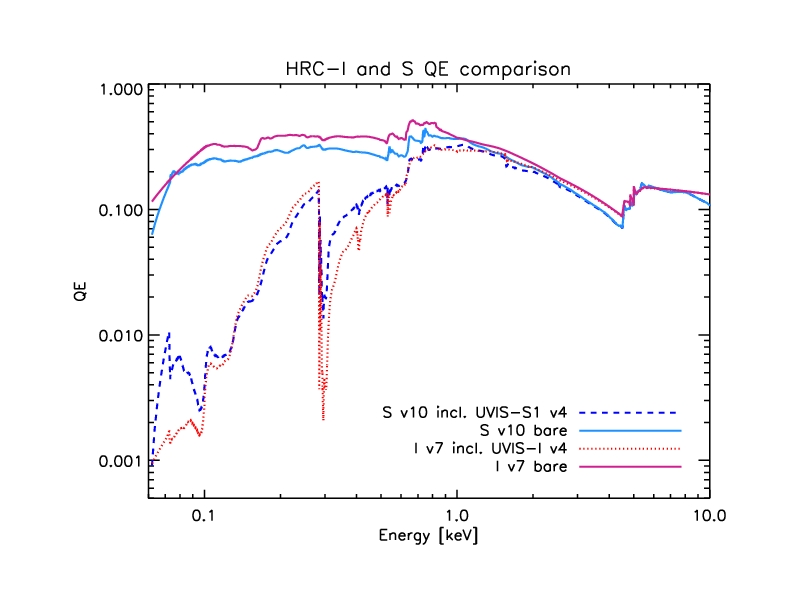Figure 1: HRC-I and S QE models with and without UVIS efficiency.

The following figure compares the UVIS-I (red) and -S1 (blue) models: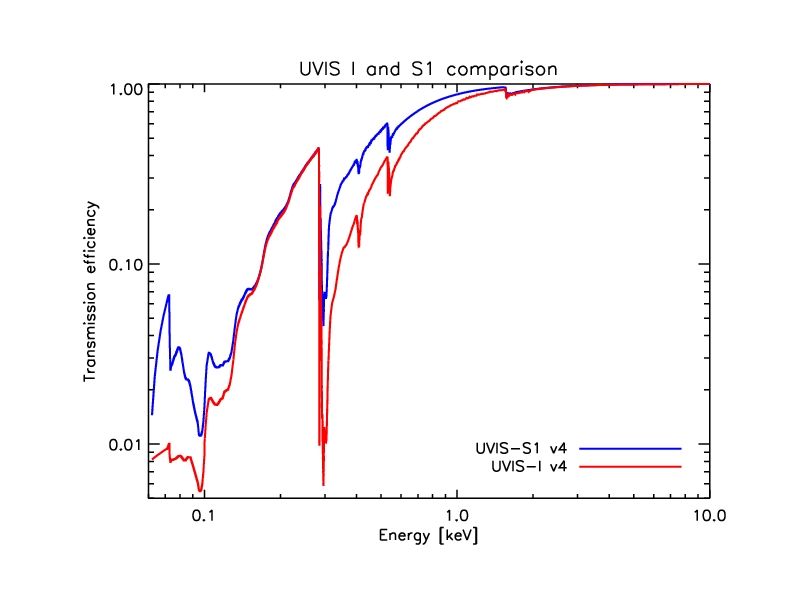Figure 2: UVIS efficiency models

Note that the "low-energy paste" will be done with the bare MCP QE models, not including UVIS efficiencies.

The following plot shows the HRC-I and S MCP QE models (pink and blue lines), with the dashed green line showing the HRC-S model raised to match the HRC-I model around 626 eV. (The red X shows the point where we will do the paste.)Figure 3: Close-up of "low-energy paste"

Model with new paste: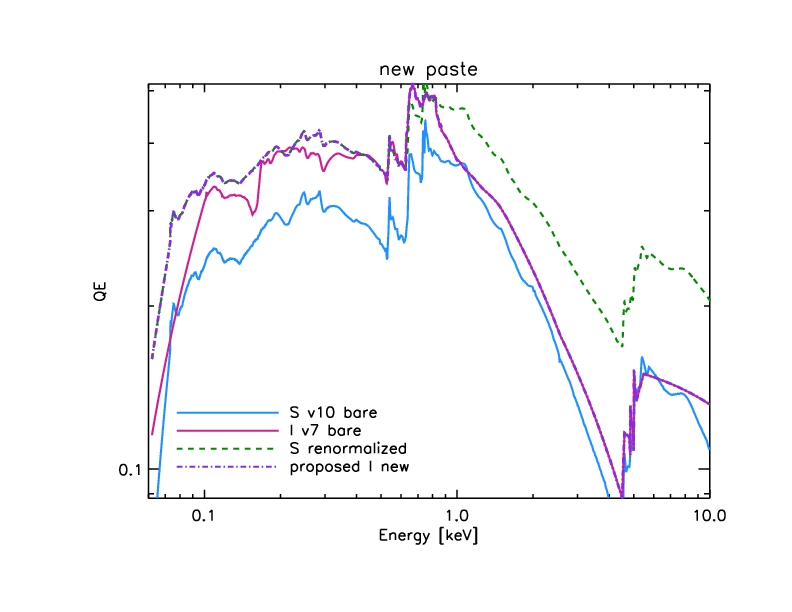Figure 4: HRC-I MCP model with new low-energy paste (purple dot-dash line).

After redoing this low-energy paste, the UVIS-I efficiency is folded back in, and the resulting QE is saved as hrciD1999-07-22qeN0007prime.fits.

### 3. Checking QE model normalization

To check the normalization of the HRC-I QE model, we use HRC-S/LETG and ACIS observations of common sources to generate source models. We use these source models and HRC-I ARFs to predict HRC-I count rates. We then compare the predicted rates to observed HRC-I count rates. We have used three calibration sources for this effort: SNR G21.5-0.9, blazar PKS 2155-304, and white dwarf HZ 43. Each of these sources are discussed in detail below. A summary of the calibration observations used in this analysis is shown in Table 1. A summary of observed count rates and predicted count rates with models N0007 and N0007prime is shown in Table 2.

#### 3.1 Hard Band: G21.5-0.9

##### 3.1.1 Observed HRC-I Count Rates
The observed count rates are taken from reprocessed level=2 event files, filtered using the ciao deflare tool to remove times affected by high background rates. Source counts are extracted from a circular region with radius 43" centered on the pulsar and background counts are taken from a large annulus with inner radius 10' and outer radius 12'.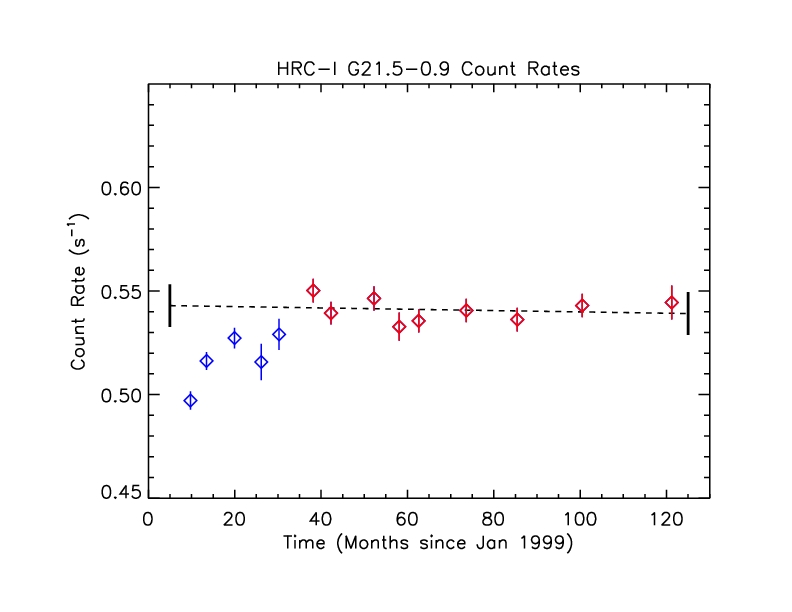Figure 5: Observed G21.5-0.9 count rates

Count rates from the first several observations are depressed for reasons we do not yet understand. For the purposes of cross-calibration to renormalize the HRC-I QE model, we do a linear fit to the points shown in red, beginning around t=40 months. The best-fit line has a slope that is consistent with zero (-3.205e-05 +/- 8.270e-05 cts/s/month) and y-intercept of 0.543 +/- 0.006 cts/s (errors are 1 sigma). The mean rate is 0.541 cts/s with standard deviation 0.006. The mean of the errors is 0.006.

##### 3.1.2 Source Model
To generate a source model for G21.5-0.9, we used three ACIS-S3 subarray observations (ObsIDs 1553, 1554 and 3693). We choose to use the subarray observations as they are not affected by pileup. The data were reprocessed with CIAO 4.2. Source counts were extracted from a circular region with radius 43" centered on the pulsar and background counts were extracted from an identical sized region located at least 165" away from the pulsar. The spectra and response files were generated using specextract We jointly fit the three spectra in XSPEC with an absorbed powerlaw model (TBabs*pegpwrlw, abund=wilm, xsect=vern) over the 1-8 keV band. The eMin and eMax parameters of the pegpwrlw model are frozen to 2.0 and 8.0 keV. The spectra, best-fit model and residuals are shown in the following plot: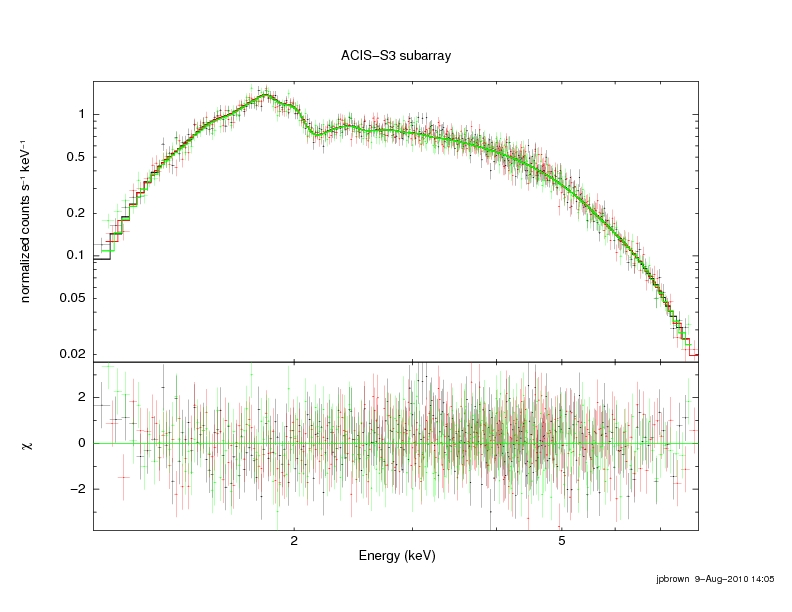Figure 5: Fit to ACIS-S3 observations of G21.5-0.9.

We obtain best-fit parameters of nH = 3.23466 x 10^22 cm^-2 [3.20203, 3.26783], PhoIndex = 1.79686 [1.78078, 1.81308] and norm = 54.9971 x 10^-12 erg cm^-2 s^-1 [54.7394, 55.2557] (90% confidence intervals indicated in brackets), with chi-squared = 969.97 using 924 PHA bins (reduced chi-squared = 1.0532 for 921 degrees of freedom).

##### 3.1.3 Predicted Rates
To get predicted count rates for G21.5-0.9 with HRC-I QE models N0007 and N0007prime we read the HRC-I ARF (made with given QE model) into XSPEC, define the source model with parameters set to values from the ACIS fit, and check the model predicted rate. For both N0007 and N0007prime, the predicted rate is 0.601 cts/s, about 11% higher than the observed rate of 0.541+/- 0.008 cts/s. The uncertainty on the predicted rate due to uncertainties in the ACIS fit is <1%, though there is ~3% scatter among the individual ACIS fluxes.

#### 3.2 Medium Band: PKS 2155-304

Since the blazar PKS 2155-304 is a variable source, we use for QE cross-calibration three consecutive observations: two HRC-S/LETG observations (ObsIDs 3709 and 4406), and an HRC-I/LETG observation (ObsID 3716) that was sandwiched between them. Absorbed power-law fits to the two HRC-S/LETG spectra give best-fit parameters which agree within 1-sigma uncertainties, so we are confident that the source flux was constant throughout the three observations.
##### 3.2.1 Observed HRC-I Count Rate
We extract counts from the reprocessed level=2 event file for ObsID 3716. Source counts are taken from a 1.5" radius circle and background counts are taken from two offset circles with radii 20", avoiding the grating dispersion and cross-dispersion spikes. The net observed 0th order count rate is 1.537 +/- 0.014 cts/s.
##### 3.2.2 Source Model
We use the HRC-S/LETG spectra to construct a source model. For each of the two observations, we smooth source and background counts from the PHA file (using PoA smoothie, which conserves flux), subtract the background, then divide the net counts spectrum by the exposure time and grating ARF. For each observation this "model" is set to zero where the ARF is less than 0.01 cm^2. The plus and minus orders are averaged, and finally the observations are averaged.

Combined HRC-S/LETG spectrum: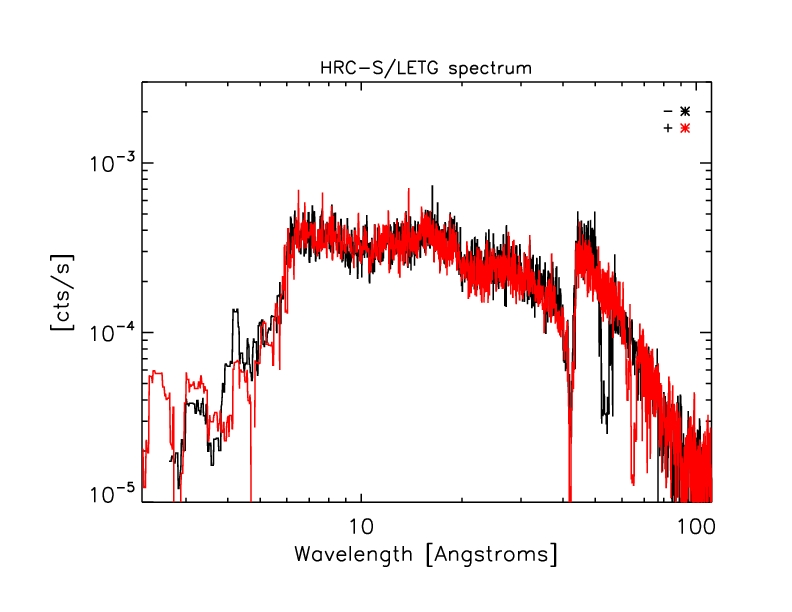Figure 6: Background-subtracted HRC-S/LETG spectrum of PKS 2155-304 (average of two observations)

The "model" (ie spectrum/ARF):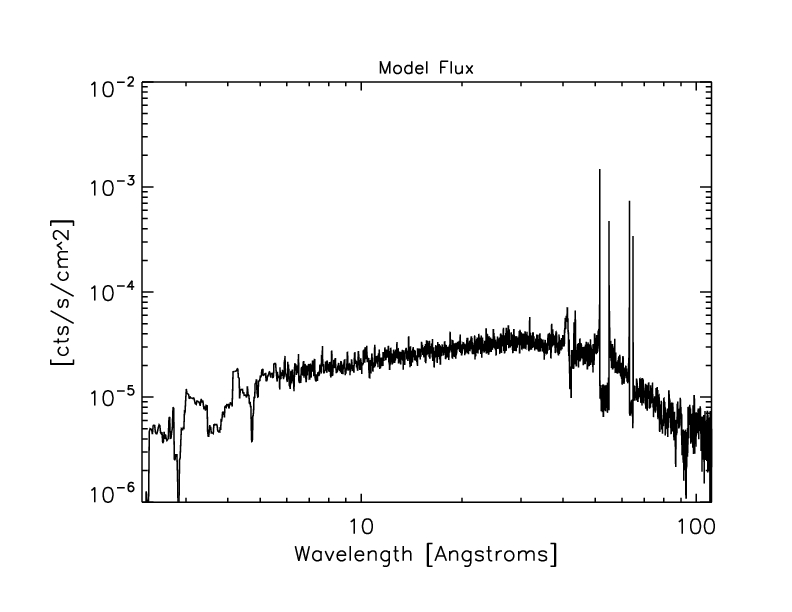Figure 7: Model derived from HRC-S/LETG spectra

The predicted HRC-I spectrum (model * HRC-I 0th order ARF):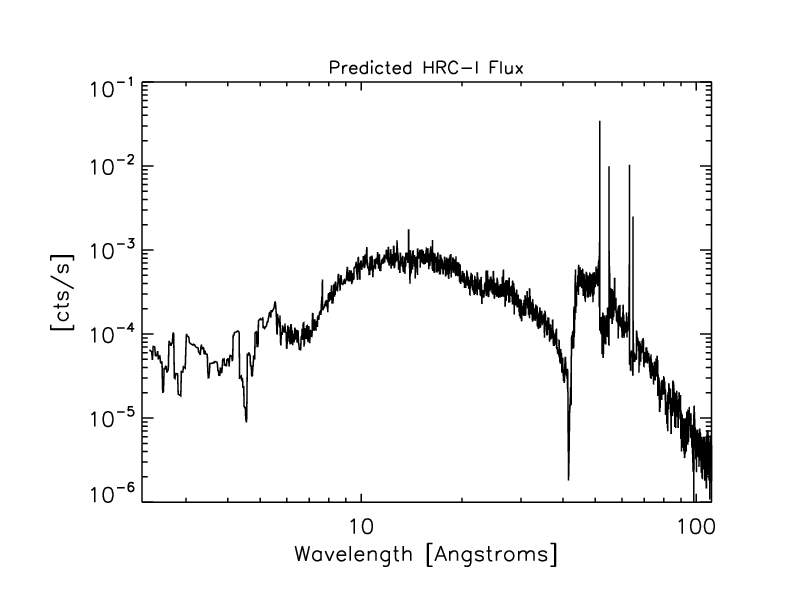Figure 8: Predicted HRC-I spectrum.

##### 3.2.3 Predicted Rate
We sum the predicted HRC-I spectrum (shown above in Figure 8) over 0.1 - 5.6 keV (where the model is > 0) to get the predicted 0th order count rate of 1.639 +/- 0.052 cts/s (6.6% higher than the observed rate) with HRC-I QE model N0007 and 1.669 +/- 0.054 cts/s (8.6% higher than the observed rate) with model N0007prime.

#### 3.3 Soft Band: HZ 43

##### 3.3.1 Observed HRC-I Count Rates
Count rates are taken from reprocessed level=2 event files which have been custom filtered to exclude times where the deadtime fraction is less than 0.985. Source rates are extracted from a circular region with radius 4.5" and background rates are taken from an annular region with inner radius 31.6" and outer radius 52.7".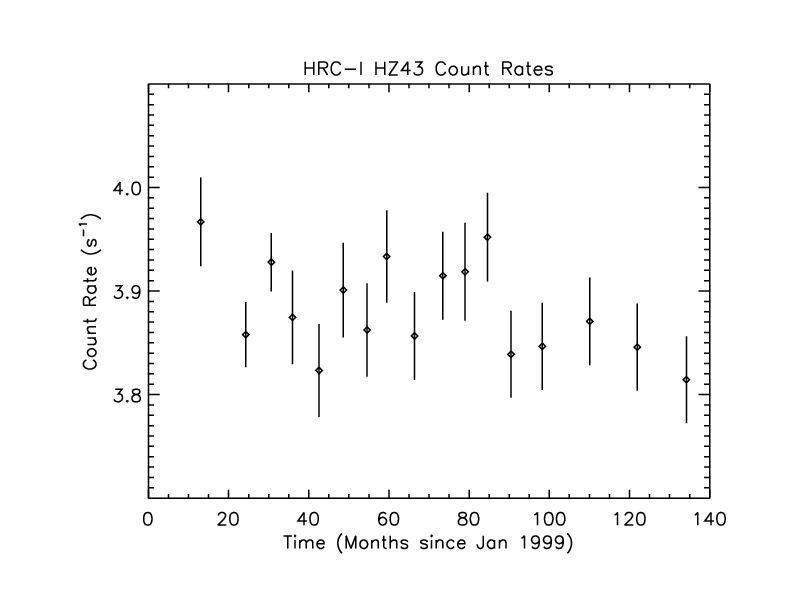Figure 9: Background-subtracted 0th order count rates for HZ43.

The mean rate is 3.88 cts/s with standard deviation 0.05. The mean of errors is 0.04.

##### 3.3.2 Source Model
As for PKS 2155-304, we construct a source model for HZ 43 using the HRC-S/LETG observations. For each observation, we smooth source and background counts from the PHA file (using PoA smoothie, which conserves flux), subtract the background, then divide the net counts spectrum by the exposure time and grating ARF. For each observation this "model" is set to zero where the ARF is less than 0.01 cm^2. The plus and minus orders are averaged, and finally the observations are averaged. However, there is a complication for this source: the observed count-rates have declined by ~9% since the initial observation due to QE decline in the HRC-S. This decline is apparent in the following plot of HRC-S/LETG 0th order count rates: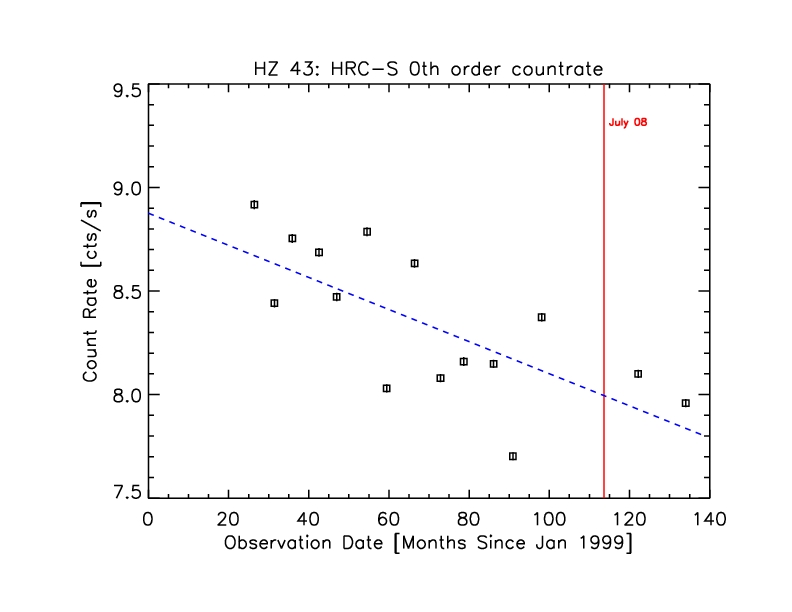Figure 10: HRC-S/LETG 0th order background-subtracted count rates with best-fit linear model shown in blue. The red vertical line marks July 2008, the epoch to which the HRC-S QE model is pinned.

To compensate for the HRC-S QE decline, we multiply the ARF for each observation by a corrective factor based on the linear fit to 0th order count rates (norm=[yint + tnrm*slope]/[yint + obs_date*slope] where tnrm corresponds to July 2008) when constructing the HZ 43 "model".

The combined HRC-S/LETG spectrum: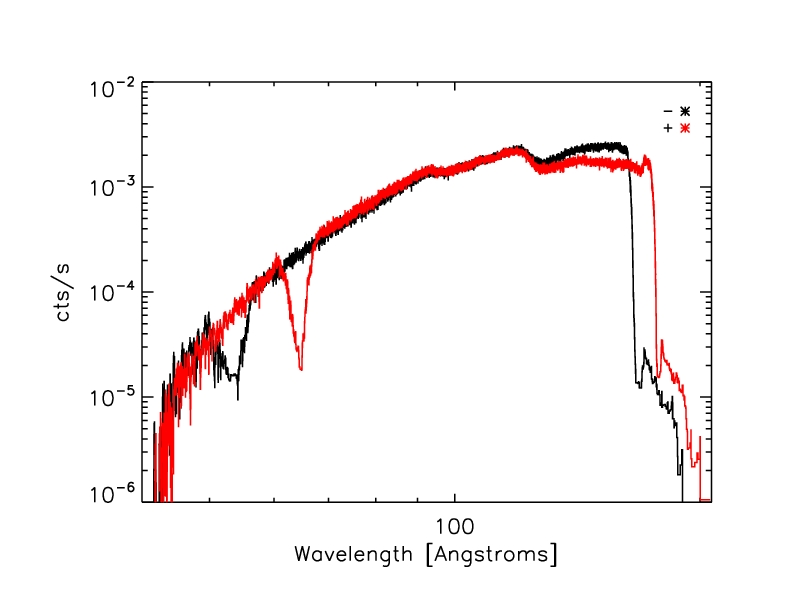Figure 11: Combined HRC-S/LETG spectrum

The "model" (ie spectrum/ARF):Figure 12: HZ 43 Model

The predicted HRC-I spectrum (model * HRC-I ARF):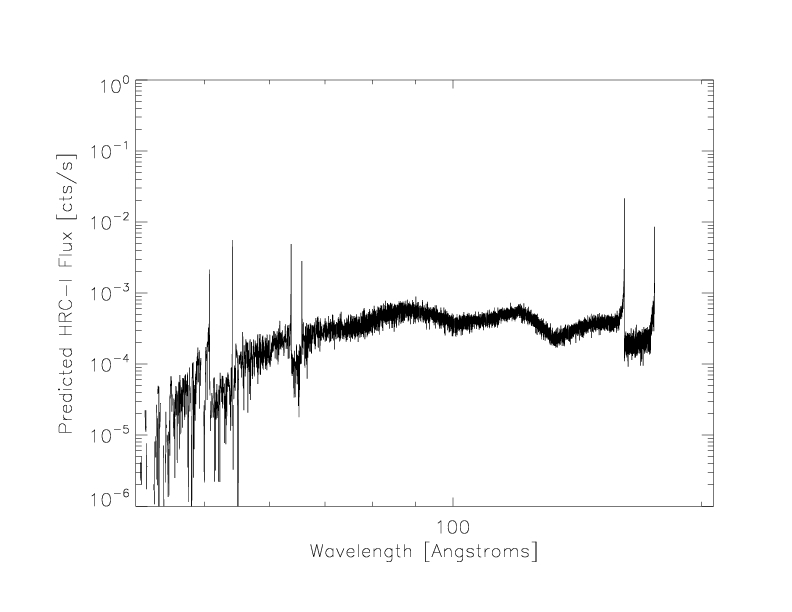Figure 13: Predicted HRC-I spectrum

##### 3.3.3 Predicted Count Rate
We sum the predicted HRC-I spectrum (shown above in Figure 13) over 0.06 - 0.3 keV to get a predicted 0th order count rate of 3.46 +/- 0.21 cts/s (10.8% below the observed rate) with HRC-I QE model N0007 and 4.03 +/- 0.25 cts/s (3.9% above the observed rate) with model N0007prime.

Table 1: Summary of calibration observations used for QE analysis.
SourceInstrumentObsIDDateExposure (s)
G21.5-0.9ACIS-S315532001-03-189741.1
15542001-07-219060.7
36932003-05-169783.6
HRC-I28672002-03-1218438.0
28742002-07-1519762.4
36942003-05-1517055.9
37012003-11-0912846.9
51672004-03-2518984.0
60722005-02-2619018.7
67422006-02-2119134.6
83732007-05-2519983.5
106482009-02-1710054.2
PKS 2155-304HRC-S/LETG37092002-11-3013741.8
44062002-11-3013762.8
HRC-I/LETG37162002-11-307328.6
HZ 43HRC-S/LETG10112001-03-1818774.9
10122001-08-1820807.7
25842002-01-0119004.0
25852002-07-2319989.8
36762002-12-0420716.5
36772003-07-2420008.8
50422003-12-2021377.1
50442004-07-1919693.1
59572005-02-0221371.7
59592005-07-2918180.1
64732006-03-1320901.2
64752006-08-0721771.7
82742007-03-1420189.3
106222009-03-1820292.0
119332010-03-1520365.9
HRC-I/LETG15142000-02-032159.6
10002001-01-123891.8
10012001-07-254955.0
26002002-01-021893.4
26022002-07-231893.3
37142003-01-241868.7
37152003-07-241894.1
50432003-12-201980.8
50452004-07-192145.3
59582005-02-202171.4
59602005-08-081758.9
64742006-01-252154.1
64762006-07-242177.8
82752007-03-192173.7
96192008-03-142164.9
106232009-03-112177.4
119342010-03-202176.0

#### 4. Renormalize N0007prime to get new QE, N0008

##### 4.1 Below 0.62 keV
First we renormalize the "re-pasted" section of model N0007prime, i.e. below 0.62 keV, based on predicted and observed HZ 43 count rates and on the PKS 2155-304 spectra/ARF ratios for this energy range.

The observed rate for HZ 43 is 3.88 +/- 0.07 cts/s and the predicted rate with N0007prime is 4.03 +/- 0.25, giving a ratio of 1.03866 with uncertainty sqrt((0.25/4.03)^2+(0.07/3.88)^2)*(4.03/3.88) = 0.0671025.

For PKS 2155-304 the ratio of (HRC-I spectrum / HRC-Iprime ARF) to (HRC-S combined spectrum / HRC-S ARF) has mean 0.983 with standard deviation 0.260 in the 20-31 Angstrom range.

(HRC-I spectrum/ARF) / (HRC-S combined spectra / ARF):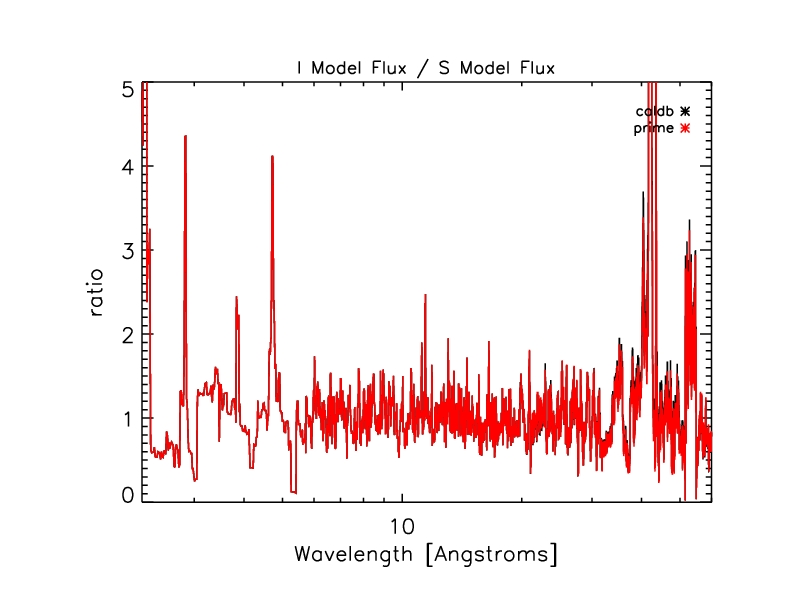Figure 14: Ratio of ARF-corrected HRC spectra for PKS 2155-304.

Close-up of range softwards of the pasting point:Figure 15: Close-up of ratio of ARF-corrected HRC spectra.

The mean value of the ratio in this range (20-31 Angstroms) is 0.983 with standard deviation 0.260.

The error-weighted mean of the I:S ARF-corrected spectrum ratios is ~1.027. So we divide the HRC-S shifted QE by 1.027 below 0.62 keV, then redo the paste to get model N0007prime_renorm. Predicted counts with this model are shown in Table 2.

New paste:Figure 16: QE paste with correct low-energy normalization.

Closeup: (The pasting point is just below 0.63 keV)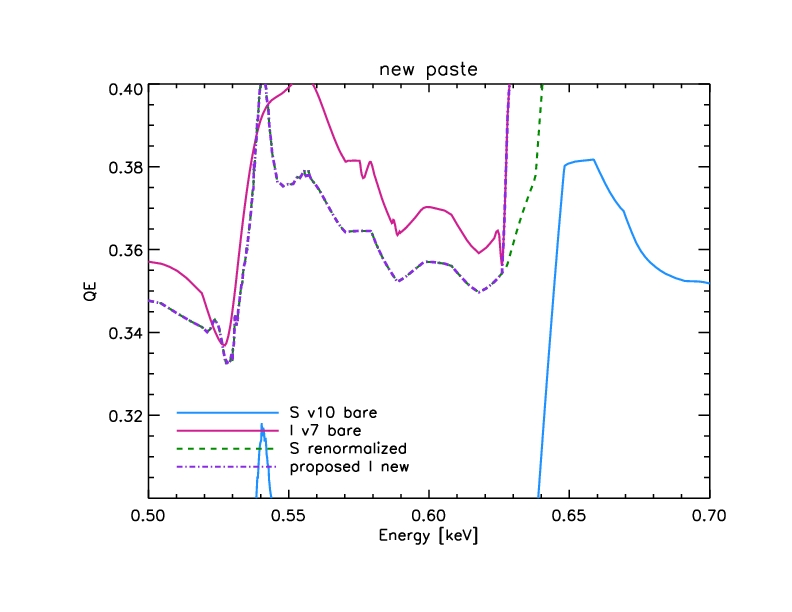Figure 17: Close-up of QE paste with correct low-energy normalization.

With this new model (N0007prime_renorm) the ratio of I to S ARF-corrected spectra for PKS 2155-304 has mean 1.01 and standard deviation 0.267 in the 20-31 Angstrom range.

##### 4.2 Large-scale correction
Finally, we do a large-scale, energy-dependent correction to model N0007prime_renorm to get the new HRC-I QE model N0008. For the correction function, we use a step function convolved with a Gaussian:

f(E)= (K/2) * (1 - Erf[ (E - E_s) / (sqrt(2) * sigma) ] + constant

where

K = amplitude of step function
E_s = energy where step is located
sigma = Gaussian sigma
constant = constant offset

We chose this function since it has the simplicity of a step function but is continuous to avoid introducing any artificial edges in the QE model.

We fix sigma to a narrow value of 0.1 keV and constrain the value of the constant so that f(0)=1.

To find values of K and E_s, we do a grid search, computing the predicted count rates for G21.5-0.9, PKS 2155-304 and HZ 43 for each pair of values by multiplying f(E) with the source model and ARF (made with HRC-I QE model N0007prime_renorm). The values yielding the minimum chi-square (0.25) are E_s = 0.35 keV and K=0.099.

The correction function: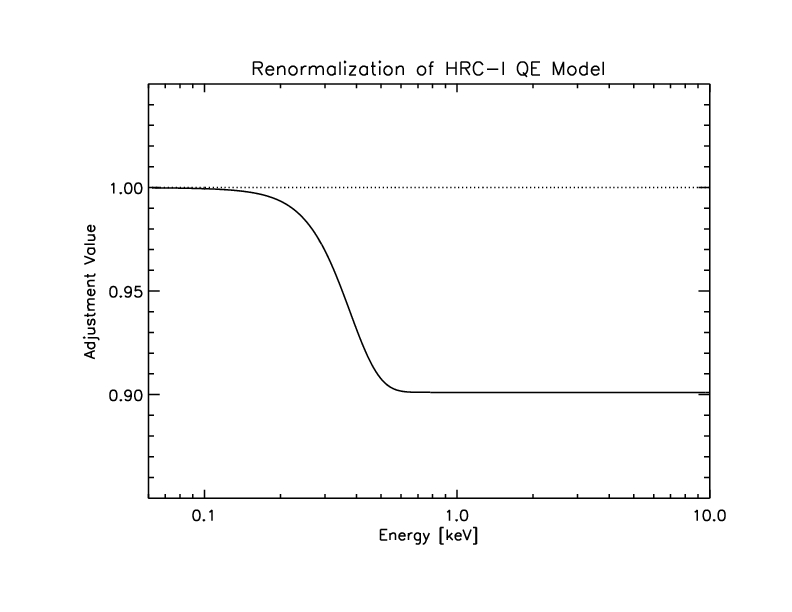Figure 18: Correction function applied to model N0007prime_renorm to get model N0008.

We multiply this function with model N0007prime_renorm to get the new HRC-I QE model N0008.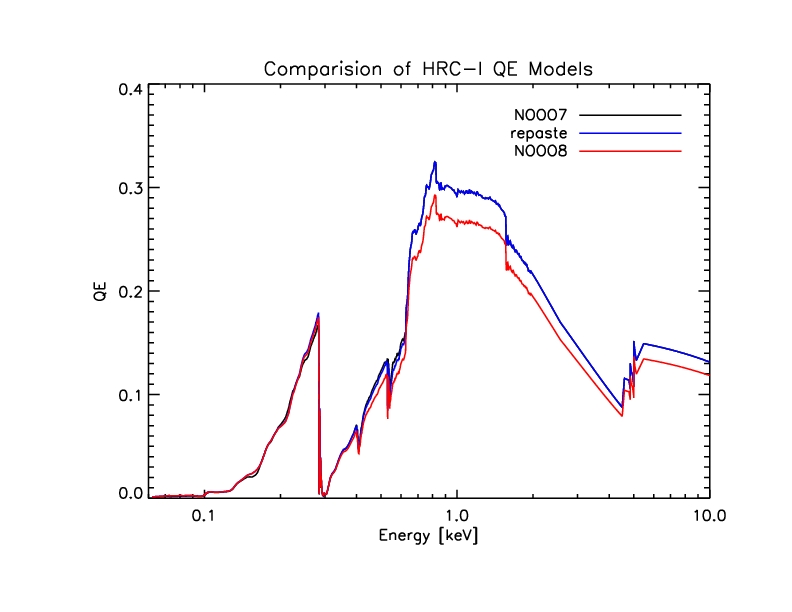Figure 19: Comparison of HRC-I QE models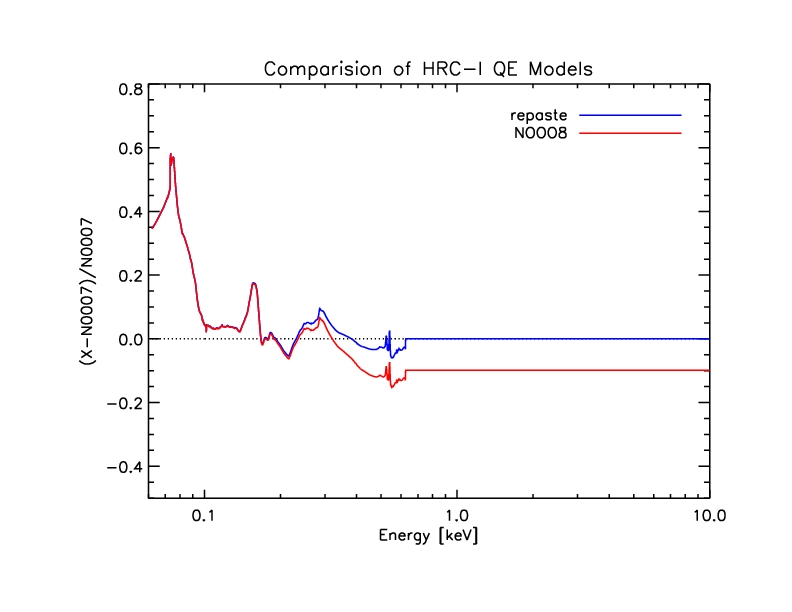Figure 20: Ratio of new (N0008) and intermediate QE models to old (N0007) QE model.

#### 5. Summary

We have updated the HRC-I QE model by adjusting the low energy shape and the global normalization. We use observations of G21.5-0.9, PKS 2155-304 and HZ 43 to cross-calibration with the HRC-S/LETG and ACIS. The mean squared error (including bias and combined variance from data and model uncertainties) is given in the last row, signifying the contribution of calibration uncertainty to predicted count rates. The uncertainty at low energies is approximately 6%, and at medium and high energies it is approximately 3%. The new HRC-I QE model (N0008) was released in the December 2010 CALDB.

Table 2: Summary of observed and predicted count rates
SourceObserved Count RatePredicted Count Rate
with N0007with N0007primewith N0007prime_renormwith N0008
G21.5-0.90.541+/- 0.0080.601 +/- 0.018
(+9.98% +/- 3.00%)
0.601 +/- 0.018
(+9.98% +/- 3.00%)
0.601 +/- 0.018
(+9.98% +/- 3.00%)
0.541 +/- 0.016
(0% +/- 2.96%)
PKS 2155-3041.537 +/- 0.0141.639 +/- 0.052
(+6.22% +/- 3.17%)
1.669 +/- 0.054
(+7.91% +/- 3.24%)
1.644 +/- 0.052
(+6.51% +/- 3.16%)
1.535 +/- 0.052
(-0.13% +/- 3.39%)
HZ 433.88 +/- 0.073.46 +/- 0.21
(-12.50% +/- 6.07%)
4.03 +/- 0.25
(+3.72% +/- 6.20%)
3.92 +/- 0.24
(+1.02% +/- 6.12%)
3.91 +/- 0.24
(+0.77% +/- 6.14%)
MSE0.2430.1140.0870.066

#### Appendix: Checking the cross calibration with E0102

To check the HRC-I / ACIS-S cross calibration, we use observations of the supernova remnant 1E0102-72.3 ("E0102"). E0102 has been observed twice with the HRC-I, in Oct 1999 and Mar 2010, and numerous times with ACIS over the course of the Chandra mission. Note that this calibration source was not used in the construction of the N0008 or preceding HRC-I QE models.

From the two HRC-I observations, we get background-subtracted source count rates of 3.22 +/- 0.01 cts/s (ObsID 1410, 1999-10-25) and 3.20 +/- 0.01 cts/s (ObsID 11093, 2010-03-04). The errors given are 1 sigma.

To calculate a predicted count rate for the HRC-I, we use as a source model a joint fit to two ACIS-S3 subarray observations (ObsIDs 3545 and 6765) with the IACHEC E0102 model. (See the Plucinsky et al 2008 SPIE paper for more information about this model.) The ACIS data are fit between 0.3 - 2 keV and the ACIS ARF includes contamination model N0007. The ACIS spectra and HRC-I counts are extracted from a circular region with radius 25.3" centered at J2000 coordinates (R.A., Dec.) = (01:04:01.996, -72:01:53.44).

From this source model and an HRC-I ARF (using HRC-I QE model N0008), we get a predicted count rate of 3.16 +/- 0.01 cts/s. The uncertainty on the predicted rate is taken from the standard deviation of 5000 MCMC iterations, sampling among the errors on the free parameters in the ACIS fit.